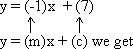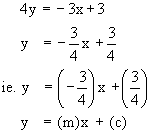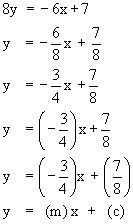Home MonkeyNotes Printable Notes Digital Library Study Guides Study Smart Parents Tips College Planning Test Prep Fun Zone Help / FAQ How to Cite New Title Request

 Intercepts on the axes We say that the line y = mx + c intersects the y - axis, at the point (0,C) Justification: any point on the y - axis has x - coordinate = 0. Since the line crosses y - axis, the point of intersection of the line y = mx + c and y - axis has x - co-ordinate = 0 putting x = 0 in y = mx + c we get y = c. Now we can say that the line y = mx + c makes an intercept c on the y-aixs. Hence for finding an intercept made on the y - axis by any line, we must put x = 0 in the equation and find y. Similarly for finding x - intercept of a line put y = 0 in the equation of the line and find x. Example Find the intercept made by the line 2y - 3x = 7 on both the coordinate axes. Solution : Putting x = 0 in 2y - 3x = 7 we get 2y = 7 \ y = 7/2 which means the line makes an intercept 7/2 on the y-axis Putting y = 0 in 2y - 3x = 7 we get -3x = 7 \ x = -7/3 Which mean the line makes an intercept -7/3 on the x - axis. Slope and intercept from (equation) of line Any straight line expressed in this form always takes the form y = mx + c where m stands for the slope and c stands for the y - intercept of the line which involves two relationships. The slope The intercept i.e. the point at which the line crosses the y - axis. When an equation is put in the form y = mx + c it is said to be in y - form or slope - intercept form. Example State the slope and y - intercept of the straight line y = -x + 7 Solution :    y = -x + 7 comparing it with y - form as y = mx + c i.e.slope m = -1 and y - intercept (c) = 7 Example    Show that the lines given by 3x + 4y -3 = 0 and 6x + 8y -7 = 0 are parallel. Solution : Ist line is 3x + 4y - 3 = 0 Putting in y - form, we get,Therefore slope m1 = -3/4 Also, the second line is 6x + 8y -7 = 0 putting in y - form, we get,Therefore, slope m2 = -3/4 Since m1 = m2, the two lines are parallel Thus when an equation is put in the form y = mx + c i.e. y - form, it should be evident that the slope is the same as the coefficient of x term. Index 8.1 Definition8.2 Relations, Graphs and Symmetry8.3 Slopes and Intercepts Chapter 9
 Search: All Products Books Popular Music Classical Music Video DVD Toys & Games Electronics Software Tools & Hardware Outdoor Living Kitchen & Housewares Camera & Photo Cell Phones Keywords: